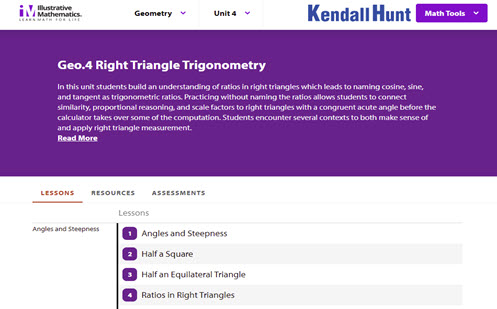Go to website

# Right-angle triangle trigonometry

In this unit students build an understanding of ratios in right triangles, which leads to naming cosine, sine and tangent as trigonometric ratios. Students encounter several contexts to both make sense of and apply right-angle triangle measurement.

Year level(s) Year 9
Audience Teacher
Purpose Student task, Teaching resource, Content knowledge
Format Web page
Teaching strategies and pedagogical approaches Explicit teaching
Keywords Pythagoras, Hypotenuse, Adjacent, Opposite, Tangent, Cosine, Sine, Angle, Ratio, Similar, Trigonometry, Right-angled triangle

## Curriculum alignment

Curriculum connections Critical and creative thinking, Numeracy
Strand and focus Space
Topics Pythagoras and trigonometry
AC: Mathematics (V9.0) content descriptions
AC9M9SP02
Apply the enlargement transformation to shapes and objects using dynamic geometry software as appropriate; identify and explain aspects that remain the same and those that change

AC9M9M03

Solve spatial problems, applying angle properties, scale, similarity, Pythagoras' theorem and trigonometry in right-angled triangles

Numeracy progression Proportional thinking (P7)
Understanding geometric properties (P7)
Understanding units of measurement (P10)